Scilab Examples – 2D plots

This is a practical approach to plots in Scilab. Several examples are shown to explore the capabilities of this software. After each line of code is explained, we show the result produced.

Simplest forms

Function plot2d plots a set of 2D curves. If you are familiar with the Matlab plot syntax, you could use function plot. If x and y are vectors, plot2d(x, y, <opt_args>) plots vector y versus vector x. x and y vectors must have the same size.

x = 0 : .1 : 10*%pi;
y = x .* cos(x);
// Plot as used in Matlab
plot(x, y)
// Add title, labels and legend
title('x * cos(x)')
xlabel('x'); ylabel('y');
legend('y = x * cos(x)');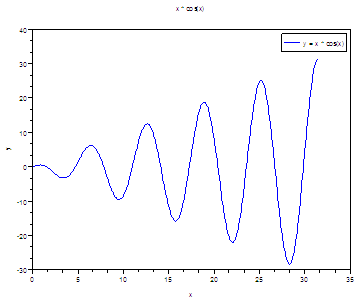// Multiple plot with captions and given tics + style
x = [0 : 0.1 : 2*%pi]';
plot2d(x, [sin(x) sin(2*x)], [2, 5],...
leg = "sin(x)@sin(2x)", nax = [2, 5, 1, 5],...
rect = [0, -1, 2*%pi, 1])

// [2, 5] means lines in blue (2) and red (5)
// leg = "sin(x)@sin(2x)" are the legends of the lines
// nax = [2, 5, 1, 5] are the tics and subtics of the
// horizontal and
// vertical axis
// rect = [0, -1, 2*%pi, 1] are the bounds of the axis,
// from (0, -1) to (2pi, 1).Logarithmic Axis – Plots

t = linspace(0, 2*%pi, 300);
x = exp(-t);
y = t;
// Use the logflag option to define logarithmic or normal axis
// n for normal, l for logarithmic.
plot2d(x, y, logflag = "ln");
xgrid;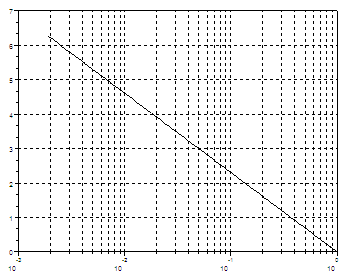// Open a new figure window with scf
scf(); plot2d(t, exp(t), logflag = "nl"); xgrid;// Both axis are logarithmic now
scf(); plot2d(exp(t), 50 + exp(2*t), logflag = "ll") ; xgrid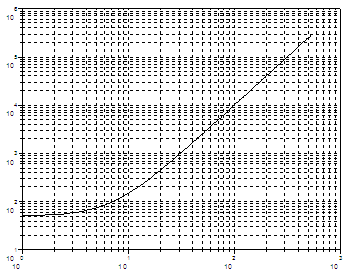Polar Plots

polarplot - Plot polar coordinates
polarplot(theta, rho, <opt_args>)

t = linspace(0, 2*%pi, 100);
r = sqrt(abs(2 * cos(5*t)));
// Plot in polar coordinates
polarplot(t, r);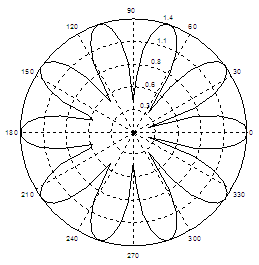Polar Plots in Matlab

Histograms in Scilab

histplot - plot a histogram
histplot(bins, data, <opt_args>)

// Define your data or get it somehow
y = 10 * rand(500, 1);
// Create the histogram. The 5 at the beginning means 5 bins.
// The 2 at the end means lines in blue.
histplot(5, y, 2)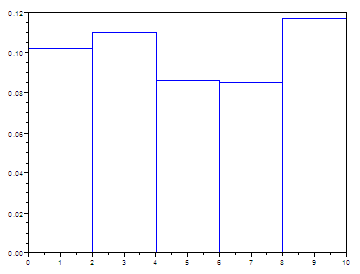Histograms in Matlab

Pie Plots

pie - draw a pie
pie(x [, sp [,txt]])

x is a scalar or a vector of real, positive numbers.
sp is a real scalar or vector.
txt means a cell or a vector of strings.

pie([1 3 4 5], [1 0 1 0],...
["Part1 Exp.","Part2","Part3 Exp.", "P4"]);

// [1 3 4 5] is the number and size of sectors
// [1 0 1 0], 1 for exploded sector, 0 for nonexploded
// ["Part1 Exp.","Part2","Part3 Exp.", "P4"] is the associated
// text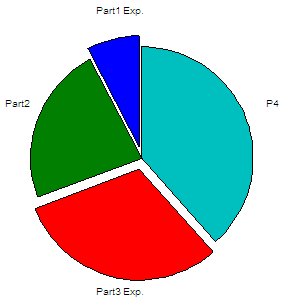Pie Plots in Matlab

Stems – Vertical lines

plot2d3 - 2D plot (vertical bars)
plot2d3([logflags,] x,y,[style,strf,leg,rect,nax])
plot2d3(x,y <,opt_args>)

plot2d3 is the same as plot2d but curves are plotted using vertical bars. By default, successive plots are superposed. To clear the previous plot, use clf().

x = [0 : 0.2 : 4*%pi];
// Define your functions and plot.
// The default color is black
plot2d3(x, sin(x))
// 5 at the end means red line
plot2d3(x, sin(2*x), 5)
// Superimpose a red envelope on the red lines
plot2d(x, sin(2*x), 5)Stems in Matlab

Stairs Function

Use plot2d2 for this purpose.

// Define your variables and functions
t = linspace(-2*%pi, 2*%pi, 200);
r = sqrt( abs(2 * cos(4*t)) );
y = r .* cos(t);
// Plot using a red line (5) and
// bound axis from (-1.5, 0) to (1.6, 1.5)
plot2d2(t, y, 5, rect = [-1.5, 0, 1.6, 1.5]);
// Add title, labels, legend and grid
title('Stairs/Steps Function');
xlabel('x'); ylabel('y');
legend('y = Step function');
xgrid;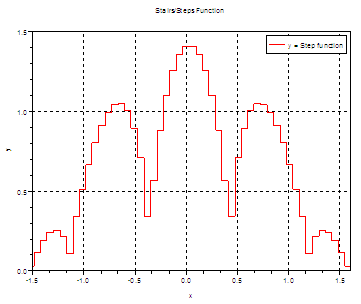From 'Scilab Examples' to Matlab home

From 'Scilab Examples' to Scilab Menu

 Top